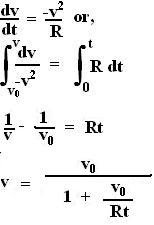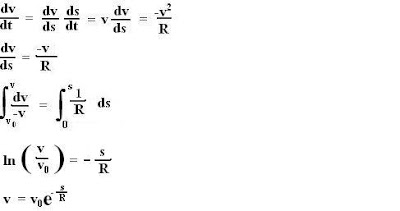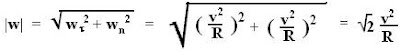## Saturday, July 21, 2007

### Irodov Problem 1.38

(a) The normal acceleration is the centripetal acceleration of the particle and is given by, v2/R, v is the instantaneous tangential velocity of the particle. Since the tangential deceleration and normal acceleration are equal, this means that the particle is decelerating tangentially at a rate v2/R.
In other words,To find the velocity v as a function of distance covered s we could use the relation between v and t to find the relation between s and t and so on... however, a simpler trick exists,(b) Once (a) is done, we already know that the tangential and normal acceleration components are equal, thus, the net acceleration must be,knowing the relation between v and s we can determine the total acceleration as a function of s as,